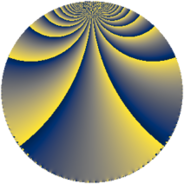# Properties

 Label 216.3.hLevel $216$ Weight $3$ Character orbit 216.h Rep. character $\chi_{216}(53,\cdot)$ Character field $\Q$ Dimension $32$ Newform subspaces $6$ Sturm bound $108$ Trace bound $4$

# Related objects

## Defining parameters

 Level: $$N$$ $$=$$ $$216 = 2^{3} \cdot 3^{3}$$ Weight: $$k$$ $$=$$ $$3$$ Character orbit: $$[\chi]$$ $$=$$ 216.h (of order $$2$$ and degree $$1$$) Character conductor: $$\operatorname{cond}(\chi)$$ $$=$$ $$24$$ Character field: $$\Q$$ Newform subspaces: $$6$$ Sturm bound: $$108$$ Trace bound: $$4$$ Distinguishing $$T_p$$: $$5$$

## Dimensions

The following table gives the dimensions of various subspaces of $$M_{3}(216, [\chi])$$.

Total New Old
Modular forms 78 32 46
Cusp forms 66 32 34
Eisenstein series 12 0 12

## Trace form

 $$32q - 2q^{4} + O(q^{10})$$ $$32q - 2q^{4} - 14q^{10} + 18q^{16} - 46q^{22} + 160q^{25} + 74q^{28} + 64q^{31} - 88q^{34} + 30q^{40} - 256q^{46} + 240q^{49} - 124q^{52} - 128q^{55} - 364q^{58} - 182q^{64} + 102q^{70} + 80q^{73} - 116q^{76} - 384q^{79} + 472q^{82} + 398q^{88} - 72q^{94} + 96q^{97} + O(q^{100})$$

## Decomposition of $$S_{3}^{\mathrm{new}}(216, [\chi])$$ into newform subspaces

Label Dim. $$A$$ Field CM Traces $q$-expansion
$$a_2$$ $$a_3$$ $$a_5$$ $$a_7$$
216.3.h.a $$2$$ $$5.886$$ $$\Q(\sqrt{2})$$ $$\Q(\sqrt{-6})$$ $$-4$$ $$0$$ $$-2$$ $$10$$ $$q-2q^{2}+4q^{4}+(-1+\beta )q^{5}+(5+\beta )q^{7}+\cdots$$
216.3.h.b $$2$$ $$5.886$$ $$\Q(\sqrt{2})$$ $$\Q(\sqrt{-6})$$ $$4$$ $$0$$ $$2$$ $$10$$ $$q+2q^{2}+4q^{4}+(1+\beta )q^{5}+(5-\beta )q^{7}+\cdots$$
216.3.h.c $$6$$ $$5.886$$ 6.0.121670000.1 None $$-1$$ $$0$$ $$-2$$ $$-10$$ $$q-\beta _{1}q^{2}+(-1+\beta _{2})q^{4}+(-\beta _{2}-\beta _{4}+\cdots)q^{5}+\cdots$$
216.3.h.d $$6$$ $$5.886$$ 6.0.121670000.1 None $$1$$ $$0$$ $$2$$ $$-10$$ $$q+\beta _{1}q^{2}+(-1+\beta _{2})q^{4}+(\beta _{2}+\beta _{4}+\cdots)q^{5}+\cdots$$
216.3.h.e $$8$$ $$5.886$$ 8.0.629407744.1 None $$0$$ $$0$$ $$0$$ $$-48$$ $$q+\beta _{1}q^{2}+(-1+\beta _{5}+\beta _{7})q^{4}+(-2\beta _{1}+\cdots)q^{5}+\cdots$$
216.3.h.f $$8$$ $$5.886$$ 8.0.$$\cdots$$.11 None $$0$$ $$0$$ $$0$$ $$48$$ $$q+\beta _{1}q^{2}+\beta _{2}q^{4}+(\beta _{1}-\beta _{5})q^{5}+(6+\cdots)q^{7}+\cdots$$

## Decomposition of $$S_{3}^{\mathrm{old}}(216, [\chi])$$ into lower level spaces

$$S_{3}^{\mathrm{old}}(216, [\chi]) \cong$$ $$S_{3}^{\mathrm{new}}(24, [\chi])$$$$^{\oplus 3}$$$$\oplus$$$$S_{3}^{\mathrm{new}}(72, [\chi])$$$$^{\oplus 2}$$